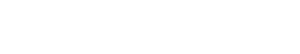# Quantile Deviation

Quantile Deviation is a statistical measure that quantifies variability in a data set. It measures the dispersion of data points around the median, and can be used to identify outliers. Quantile Deviation is also known as Median Absolute Deviation (MAD), and is sometimes referred to as Average Absolute Deviation (AAD).

There are several ways to calculate Quantile Deviation, but the most common method is to first calculate the median of the data set, and then take the absolute value of the difference between each data point and the median. The resulting values are then summed and divided by the number of data points.

Quantile Deviation can be useful for identifying outliers in data sets, as points that are far from the median are more likely to be outliers. However, it is important to note that Quantile Deviation does not identify which direction the outliers are in (e.g. above or below the median), only that they are further away from the median than other points in the data set.

## Outside of text analytics industry

Quantile Deviation is also used outside of the text analytics industry, albeit with a slightly different definition. In statistics, Quantile Deviation is defined as the difference between two specified quantiles of a data set. For example, the interquartile range (IQR) is calculated as the difference between the 75th and 25th percentile of a data set.

Similarly, the semi-interquartile range (SIQR) is calculated as the difference between the 50th and 25th percentile, or the 75th and 50th percentile.

Both the IQR and SIQR are measures of dispersion, but they differ from Quantile Deviation in that they measure dispersion around the median, rather than around the mean.

However, it is important to note that the term “Quantile Deviation” can also be used to refer to the difference between any two quantiles, not just those at the extremes (25th and 75th percentiles).

## Quantile Deviation and dispersion

While Quantile Deviation is a measure of dispersion, it should not be confused with other measures of dispersion such as standard deviation. Standard deviation measures the variability of data points around the mean, while Quantile Deviation measures the variability of data points around the median.

Another common measure of dispersion is variance, which is simply the square of the standard deviation. Like standard deviation, variance measures the variability of data points around the mean.

All three measures (Quantile Deviation, standard deviation, and variance) are used to quantify variability in data sets, but it is important to note that they are not interchangeable. Each has its own unique advantages and disadvantages, and so it is important to choose the appropriate measure for the data set and the question being asked.Unlock the power of actionable insights with AI-based natural language processing.